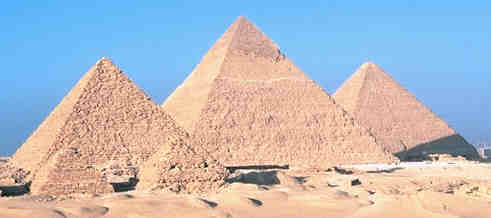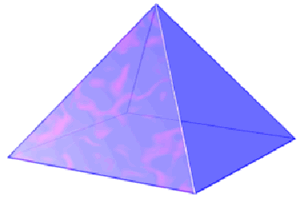# Square Pyramid

Go to Surface Area or Volume.

Square Pyramid Facts

Notice these interesting things:

• It has 5 Faces
• The 4 Side Faces are Triangles
• The Base is a Square
• It has 5 Vertices (corner points)
• It has 8 Edges

## Surface AreaWhen side faces are the same:

Surface Area = [Base Area] + 1 2 × Perimeter × [Slant Length]

### Example: Side faces are the same, slant length is 3, base is square with side length of 5

Surface Area = [Base Area] + ½ × Perimeter × [Slant Length]
= [5 × 5] + ½ × (5+5+5+5) × 3
= 25 + ½ × 20 × 3
= 25 + 30
= 55

When side faces are different we can calculate the area of the base and each triangular face separately and then add them up.

## Volume

Volume = 1 3 × [Base Area] × Height

### Example: base is square with side length of 5 , height is 6

Volume= 1 3 × [Base Area] × Height
= 1 3 × [5 × 5] × 6
= 1 3 × 150
= 50

Play with it here. Note how the formula stays the same when it "leans over" (oblique):

## Other Images